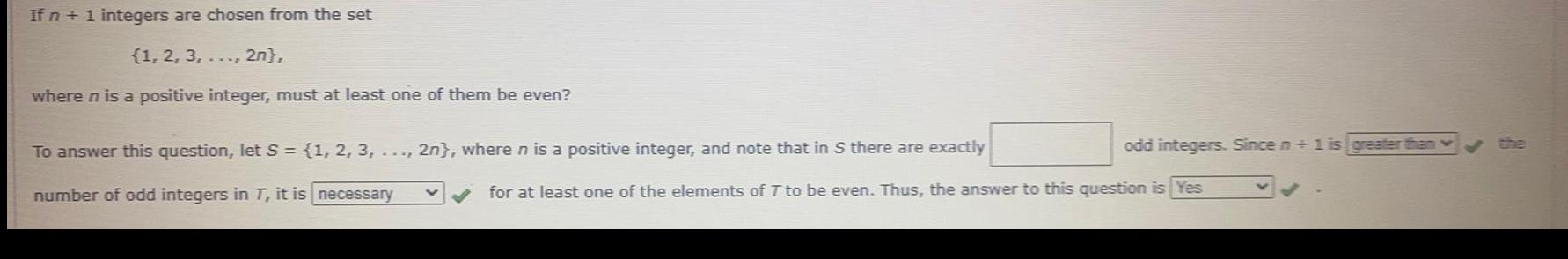Question:

# If n + 1 integers are chosen from the set {1, 2, 3,..., 2

Last updated: 8/10/2022If n + 1 integers are chosen from the set {1, 2, 3,..., 2n}, where n is a positive integer, must at least one of them be even? To answer this question, let S = {1, 2, 3,..., 2n}, where n is a positive integer, and note that in S there are exactly _____ number of odd integers in T, it is ______ odd integers. Since n + 1 is _____ v for at least one of the elements of T to be even. Thus, the answer to this question is _____